# Colleges with the highest SAT scores in Wisconsin

Top 10 colleges in Wisconsin with the highest SAT scores
Looking for the colleges with the highest SAT scores in Wisconsin? Well you're in luck! We've compiled a national college database and have created a list of the top 10 universities with the highest SAT scores in Wisconsin. These are the schools whose applicants had the highest average SAT scores in Wisconsin. And, since these tests are meant to determine academic prowess, they are arguably the schools with the most academically proficient students. You could even say these are the best colleges in Wisconsin. We also include each college's ACT scores and acceptance rate so that you can see where you would have the easiest or hardest time getting in. Read on to find out more.

## University of Wisconsin Madison SAT scores

The average SAT score for University of Wisconsin Madison is 1390.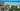The average SAT score of 1390 breaks down into:

• SAT math: 725

The average ACT score for University of Wisconsin Madison is 30 and their acceptance rate is 51.7%.

## Lawrence University SAT scores

The average SAT score for Lawrence University is 1340.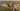The average SAT score of 1340 breaks down into:

• SAT math: 670

The average ACT score for Lawrence University is 29 and their acceptance rate is 62.5%.

## Milwaukee School of Engineering SAT scores

The average SAT score for Milwaukee School of Engineering is 1260.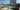The average SAT score of 1260 breaks down into:

• SAT math: 660

The average ACT score for Milwaukee School of Engineering is 27 and their acceptance rate is 62.8%.

## University of Wisconsin Platteville SAT scores

The average SAT score for University of Wisconsin Platteville is 1245.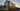The average SAT score of 1245 breaks down into:

• SAT math: 630

The average ACT score for University of Wisconsin Platteville is 24 and their acceptance rate is 79.1%.

## Marquette University SAT scores

The average SAT score for Marquette University is 1240.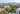The average SAT score of 1240 breaks down into:

• SAT math: 620

The average ACT score for Marquette University is 27 and their acceptance rate is 81.7%.

## Saint Norbert College SAT scores

The average SAT score for Saint Norbert College is 1210.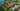The average SAT score of 1210 breaks down into:

• SAT math: 605

The average ACT score for Saint Norbert College is 25 and their acceptance rate is 77.7%.

## Carroll University SAT scores

The average SAT score for Carroll University is 1180.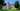The average SAT score of 1180 breaks down into:

• SAT math: 590

The average ACT score for Carroll University is 24 and their acceptance rate is 70.8%.

## University of Wisconsin La Crosse SAT scores

The average SAT score for University of Wisconsin La Crosse is 1174.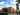The average SAT score of 1174 breaks down into:

• SAT math: 590

The average ACT score for University of Wisconsin La Crosse is 25 and their acceptance rate is 78.2%.

## Northland College SAT scores

The average SAT score for Northland College is 1170.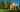The average SAT score of 1170 breaks down into:

• SAT math: 570

The average ACT score for Northland College is 23 and their acceptance rate is 61.2%.

## Wisconsin Lutheran College SAT scores

The average SAT score for Wisconsin Lutheran College is 1152.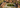The average SAT score of 1152 breaks down into:

• SAT math: 570